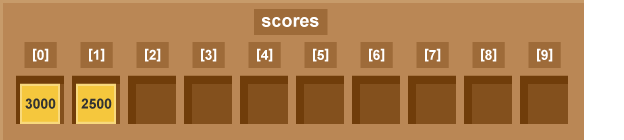Computing
Computer Science LearnITWithMrC ⛯ Year 7 Year 8 Year 9 GCSE## Lesson Video 20

•Watch the Lesson video
Make notes on your notes sheet.
•Make notes on your notes sheet.
•Complete the learning activities
Make any notes on your notes sheet.
•Check the Learning outcomes

## What do I need to Learn?

0 results forGuest
 I need to learn how to understand and use a two dimensional array as a data structures.

## Key Terms

Data structure Array List Variable Identifier

## Sequence

The most basic data structure in Python is the sequence (lists, tuples and strings). Every element in a sequence is assigned a number, which is its position or index. The first index is always zero, the second is one, and so forth.

Indexes are numbered from 0 to n-1 where n is the number of items (or characters):

``` H  e  l  l  o  ,  _  w  o  r  l  d  !
0  1  2  3  4  5  6  7  8  9 10 11 12
```

There are certain things you can do with all sequence types. These operations include indexing, slicing, adding, multiplying, and checking for membership.

## Python Lists

The list is versatile data structure that is available in Python, it is a mutable data structure, which can be written as a list of comma-separated values (items) between square brackets.

An Important thing to note about a list is that items in a list need not be of the same type.

Creating a list is as simple as putting different comma-separated values between square brackets. For example −

```list1 = ['physics', 'chemistry', 1997, 2000];
list2 = [1, 2, 3, 4, 5 ];
list3 = ["a", "b", "c", "d"]
```

Similar to string indices, list indices start at 0, and lists can be sliced, concatenated and so on.

Click on the link below to go through a python tutorial on lists. By the end you should have a basic understanding of:

• Accessing Values in Lists
• Updating Lists
• Deleting List Elements
• Basic List Operations
• Built-in List Functions & Methods:

## Exercise 1

Take a list, say for example this one:

and write a program that prints out all the elements of the list that are less than 5.

#### Extension questions:

1. Instead of printing the elements one by one, make a new list that has all the elements less than 5 from this list in it and print out this new list.
2. Ask the user for a number and return a list that contains only elements from the original list 'a' that are smaller than that number given by the user.

## Arrays

An Array is a 'sequence' type data structure, which is a series of memory locations each of which holds a single data type. All data in an array must be of the same data type.

You can think of an array like a row of cells, like the ones found in a table. Each cell represents an element:## Exercise 1

Research the differences between an array and a list:

## Exercise 2

Write a program that first ouputs the length of a list,(you will need to create a list first, then asks the user to choose an element from that list and then prints out the value of that element

e.g. For the list:

` [1, 2, 4, 7, 11]`
` 'The list has 5 elements'`
Then ask the user to chose an element. If a users enters the number 3

Your program will return the third value: 4

#### Extension question:

1. Write a program that creates an array of all of the positive odd elements that are less than n, where n is the user input.

e.g. So if a users enters the number: 10 your program will return :

` [1, 3, 5, 7, 9]`

## Multi-Dimensional Arrays

Watch the video below for a brief introduction on how to create multi-dimensional arrays

### Exercise

Copy the following code into python and then run it to see if you can understand how it is manipulating a two dimensional array/list.

```
a = [[1, 2, 3], [4, 5, 6]]
print(a)
print(a)
b = a
print(b)
print(a)
a = 7
print(a)
print(b)
b = 9
print(a)
print(b)
```

To process a 2-dimensional array/list, you will most often use nested loops. The first loop iterates through the row number, the second loop runs through the elements inside of a row, which you can think of like a column in a table.

```a = [[1, 2, 3, 4], [5, 6]]
b = [[3, 7, 2, 7], [5, 4]]
```

#### Extension questions:

1. Write a nested loop so that it prints out all of the elements of the two-dimensional array 'a', in a table like structure
2. Find the index of the first occurrence of the maximum element in list 'b'. Print two numbers: the row number and the column number where the largest element in a two-dimensional array is.
• If there is more than one element, print the one with smaller row number. If there are many maximums with the smallest row number, output the one which has the least column number.
3. Chess board - Given two numbers n and m. Create a two-dimensional array of size n×m and populate it with the characters "." and "*" in a checkerboard pattern. Top left corner should have ".".

Click the button below for a solution to task 1.